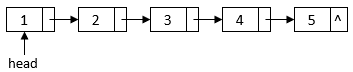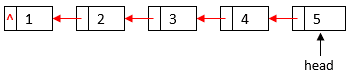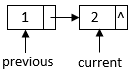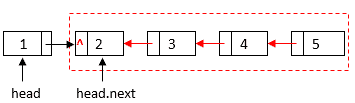## 1. Introduction

In Computer Science, a linked list is a linear data structure in which a pointer in each element determines the order. In this tutorial, we’ll show how to reverse a linked list.

Each element of a linked list contains a data field to store the list data and a pointer field to point to the next element in the sequence. We can use apointer to point to the start element of a linked list:After we reverse the linked list, thewill point to the last element of the original linked list, and the pointer of each element will point to the previous element of the original linked list:## 3. Iterative Solution

Firstly, let’s solve a simpler version of the problem: reverse a linked list with two elements:Suppose thepointer points to the second element and thepointer points to the element before theelement, we can switch the link order between them with two operations:==For a linked list with more than two elements, we can traverse the linked list and use the same strategy to reverse the current element’s next pointer:In this iterative algorithm, we first set thepointer as apointer and theas the. Then, in each iteration of the loop, we reverse the linked pointer of these two elements and shift theandpointers to the next two elements. In the end, thepointer will point to the new head element of the reversed linked list.

Since each element only has one reference to the next element, we need another pointer,,  to store the next element before changing thepointer.

The loop traverses the whole linked list once. Therefore, the running time of the iterative algorithm is, whereis the total number of elements of the linked list.

## 4. Recursive Solution

We can also solve the problem with a recursive solution. Let’s first consider a simpler case where we have reversed the rest of the linked list after theelement:We only need to reverse two elements:and. At the beginning, thepointer of theis. We should change that to make it point to. Then, we need to change the next pointer ofelement toto finish the reversal:==We can extend this solution to a recursive algorithm of reversing a linked list staring with aelement. Firstly, we can reverse the linked list starting withelement by recursively calling our reversal function.  Then, the linked list becomes our simpler case. Therefore, we can reverse the last two elements,and, with the above two operations.

We can construct a recursive algorithm based on this approach:In this recursive algorithm, we first check base cases where the inputpointer isor points to a single element. Then, we recursively call thefunction on theelement to reverse the rest of the linked list. Finally, we reverseandelements to finish the reversal.

The recursive algorithm also traverses the whole linked list once. Therefore, the running time is, whereis the total number of elements of the linked list.

## 5. Conclusion

In this tutorial, we showed a sample linked list and its reversal. Also, we discussed two algorithms that can reverse a linked list in linear time.